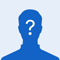# Can someone help me with these old homework questions I got wrong?1. A wind turbine is spinning at an angular speed of 6 rad/s. Each blade of the turbine is 2m long.

What is the tangential speed of a point at the tip of a blade?

2m/s

3m/s

6m/s

12m/s

I chose 6m/s but got it wrong

2. A Compact disk spinning at 30 rev./min, when the disk player is turned off. It takes 2 minutes to the disk to come to a complete stop. Find the angular displacement of the disk.

30 rev

188 rev

60 rev

I chose 60 rev but calculated it wrong

3. A ball of mass m1 moving at the velocity of +5m/s hits a second ball (which is stationary) of mass m2. After the collision, the velocity of m1 is found to be -2m/s. Select the correct answer from the following (assume that the collision is elastic).

2m1=5m2

5m1=2m2

7m1=3m2

m1=m2

I chose A but got it wrong

please explain clearly the answer and how to get to it. I can't study old homework questions that are wrong. I would appreciate it a lot
Can someone help me with these old homework questions I got wrong?
0
1